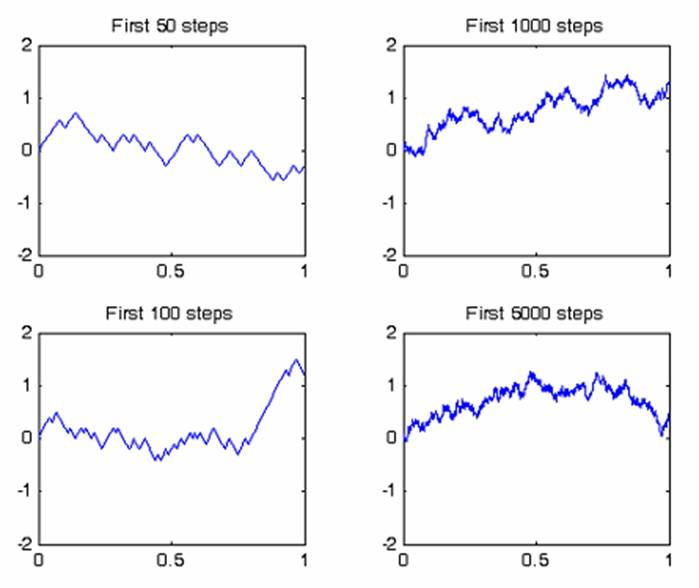Since we are all sitting in front of computer screens, it is worth talking a bit about how Brownian motion is studied in these machines!

Technically, one can say that the numerical construction of a Brownian motion can be done in two ways

·                   As a scaled random walk

·                   In terms of random Fourier series

WHAT???

OK, you are right to be complaining, this sounds confusing!

Let’s just try to understand the first of the two alternatives.

I’ll first give you the definition and then the recipe and the results.

Definition:

Brownian motion may be considered as a scaled version of the usual random walk on the lattice with probability ½ to make aleft move and ½ to make a right move. In the limit where the jumps take place every dt and the length of the jumps are dx, where dt goes to 0, dx goes to 0 AND dx2/dt goes to 1, using (something called) Central Limit Theorem, we may show that this random walk tends to the Brownian motion.

OK, but how do we actually do it?

The following short algorithm solves this problem

for i=1:N

dW(i)=sqrt(dt)*rand

W(i+1)=W(i)+dW(i)

end

Let’s see what the algorithm means: dW(i) contains the increments of the Brownian motion, rand is a random number and W(i) contains the Brownian motion at time interval “i”.

Now let’s see what we get!Voila!